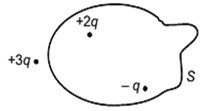Search
•CH.PHYSICS

# Important questions class 12 physics electric charges and fields

Updated: May 30, 2020

## [1 Mark Questions]

1. Why do the electrostatic field lines not form a closed-loop?

2. Why do the electric field lines never cross each other?

3. Why must the electrostatic field be normal to the surface at every point of a charged conductor?

4. Why must electrostatic field at the surface of a charged conductor be normal to the surface at every point? Give reason.

5. Two-point charges q1and q2are placed at a distance 'd’ apart as shown in the figure. The electric field intensity is zero at the point P on the line joining them as shown. Write two conclusions that you can draw from this.6. Define the dipole moment of an electric dipole. Is it a scalar quantity or a vector quantity?

7. Draw a plot showing the variation of an electric field (E) with distance r due to a point charge Q.

8. A proton is placed in a uniform electric field directed along the positive X-axis. In which direction will it tend to move?

9. In which orientation, a dipole placed in a uniform electric field is in

i. stable and

ii. unstable equilibrium?

10. Two-point charges having equal charge separated by 1 m distance experience a force of 8 N. What will be the force experienced by them if they are held in the water at the same distance? (Given K water = 80).

11. A metallic sphere is placed in a uniform electric field as shown in the figure. Which path is followed by electric field lines and why?12. Point out right or wrong for the following statement.

The mutual forces between two charges do not get affected by the presence of other charges.

13. A dipole of dipole moment p is present in a uniform electric field E. Write the value of the angle between p and E for which the torque experienced by the dipole, is minimal.

14. Consider two hollow concentric spheres, S1 and S2, enclosingcharges2Qand 4Qrespectively as shown in the figure.(i) Find out the ratio of the electric flux through them.

(ii) How will the electric flux through the sphere S1changeif a medium of dielectric constant ‘εr’ is introduced in the space inside S1inplace of air? Deduce the necessary expression.

15. Two charges of magnitude – 2Q and + Q are located at points (a, 0) and (4a, 0), respectively. What is the electric flux due to these charges through a sphere of radius 3awith its centre at the origin?

16. A charge q is placed at the centre of a cube of side l. What is the electric flux passing through each face of the cube?

17. The Figure shows three-point charges, + 2q, - q and +3q. Two charges+2qand – q are enclosed within a surface ‘S’. What is the electric flux due to this configuration through the surface ‘S’?18. Define electric flux. Write its S.I. units.

19. A charge q is enclosed by a spherical surface of radius R. If the radius if reduced to half, how would the electric flux through the surface change?

20. State the Gauss law of electrostatics.

## [3 Mark Questions]

1. Two-point charges +q and – 2q are placed at the vertices B and C of an equilateral triangle ABC of the side as shown in the figure. Obtain the expression for

i. The magnitude and

ii. The direction

Of the resultant electric field at the vertex A due to these two charges.2. Define the term electric dipole moment. Is it a scalar or vector? Deduce an expression for the electric field at a point on the equatorial plane of an electric dipole of length 2a.

3. Sketch the pattern of electric field lines due to

i. A conducting sphere having a negative charge on it.

ii. An electric dipole.

4. A positive point charge (+q) is kept in the vicinity of an uncharged conduction plate. Sketch electric field lines originated from the point on to the surface of the plate.

5. Using Gauss law, obtain the expression for the electric field due to uniformly charged spherical shell of radius R at a point outside the shell. Draw a graph showing the variation of the electric field with r, for r > R and r < R.

6. Use Gauss’ law to derive the expression for the electric field between two uniformly charged parallel sheets with surface charge densities and -, respectively.

7. State Gauss’ law in electrostatics. Using this law, derive an expression for the electric field due to a uniformly charged infinite plane sheet.

8. State Gauss’ law in electrostatics. Using this law derive an expression for the electric field due to a long straight wire of linear charge density λ C/m.